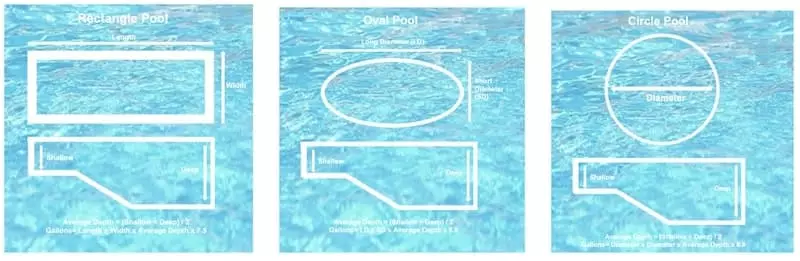## Pool pump - What size pump do I need?

In this article we will determine which size swimming pool pump is required for your swimming pool.### What happens if I buy the wrong size swimming pool pump?

There is a tendency for pool owners to buy a swimming pool pump that is too big. This leads to higher operation costs and overpowering of your filter system.

### How do I choose the right pool pump?

As a general rule you should choose a pool pump which filters all water in your pool in an eight hour period. The below steps and calculations will help you to do this very simply.

### Step 1. How to calculate the number of gallons in your pool.

• The formula for this is: length x width x depth x 7.5 = (volume in gallons)
• To calculate the Average Depth: If the shallow end is 3 feet and the deep end is 9 feet, and assuming the slope of the pool is gradual and even, then the average depth is 6 feet
• Change metres to feet: 1 metre = 3.28 feet

Example: Rectangular shape pool

pool is 9m long x 4.5m wide x an average of 1.2m deep

pool is 29.52 feet long x 14.76 feet wide x 3.94 average feet deep (29.52 x 14.76 x 3.94)

29.52 x 14.76 = 435.71. 435.71 x 3.94 = 1716.71. 1716.71 x 7.5 = 12,875.38 gallons

The calculations for different pool shapes are below.

For a kidney shaped pool measure the both the longest width and the shortest width of the pool.

Average Depth = (Shallow + Deep) / 2

Gallons = (LW x SW) x Length x Average Depth x 3.38

###Step 2. Calculate the gallons per hour required

You now need to know how many gallons of water per hour is required to be pumped in order to clean all the water in your pool over an 8 hours period. To determine this flow rate simply divide your calculated gallons by eight.

EG: 12,875.38 gallons divide x 8 = 1609.42 gallons per hour.

### Step 3. Convert gallons per hour into gallons per minute.

Divide your previously calculated gallons per hour by 60 to determine your gallons per minute.

Using the previous sum: 1609.42 gallons per hour divided x 60 = 26.82 gallons per minute

### Step 4. Convert gallons per minute into litres per minute

Using the previous sums: 26.82 multiplied x 3.78541 = 101.5 litres per minute

### Step 5. Calculate the average feet of Head for your pool pump.

A good estimate of the average head for your swimming pool pump is to take the average run of pipe from your pool skimmer, suction lines and mains drain to your pumps installation point.

To do this calculate the length of each individual suction line. If there are three lines add the three lines together to find the total length and divide by three to get the average.

Example: Skimmer line – 8 metres, Main line – 20 metres, Filter line – 19 metres = Total length of 47 metres

Divide 47m x 3 lines = 15.66m head

### Step 5. Selecting your swimming pool pump

You have now determined you require a pump delivering a minimum 101.5 L/min with a head of 16m. In this case based on the calculations and the performance graph for each pool pump you would choose the SPP1500.

You can now select your pool pump.

For further information or help or advice dont hesitate to call and speak to one of our pump technicians or contact us by email. We're here to help!